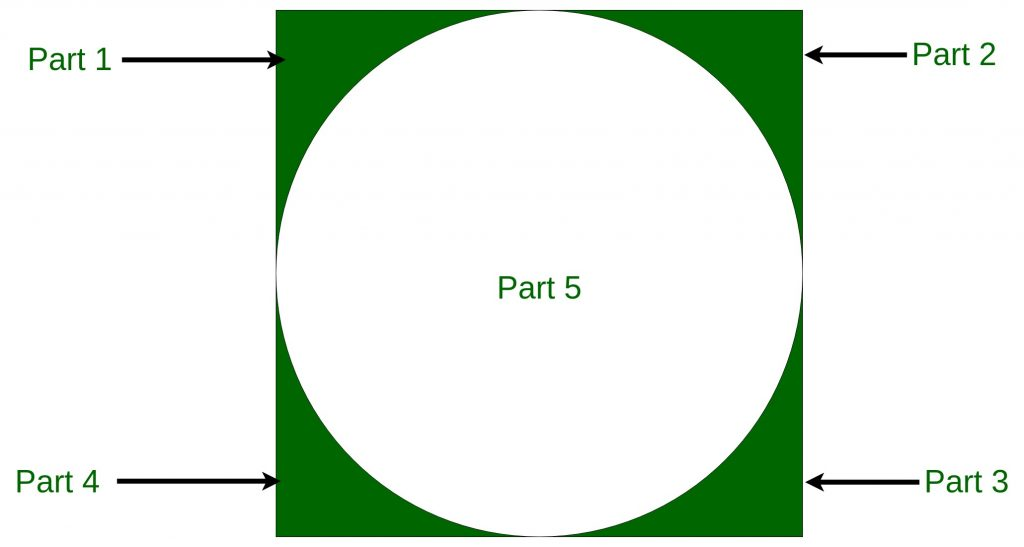Skip to content
Related Articles
Puzzle | Divide a Square into 5 parts such that 4 parts among them are equal
• Difficulty Level : Basic
• Last Updated : 17 Sep, 2019

Puzzle: We have to divide a square into 5 parts such that 4 parts among them are equal. The condition is that even once the pen does not get up.
Solution: We can solve the above puzzle in the below way, and we have to draw an inscribed circle within the square. In this way, all corner of the square are equal and square divided into 5 parts. Even if we draw a circle then we do not need to get up the pen. Draw a circle like below image:My Personal Notes arrow_drop_up